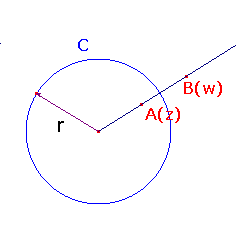Inversion in the circle C : |z| = r

 Inversion in C sends z to r2/z* (for non-zero z), and interchanges 0 and Ñ. Proof Take z non-zero, and let A be the point with complex coordinates z, and suppose that A has inverse B (coordinates w) with respect to C. By the definition of inverse points, B lies on the ray OA, so w = kz, with k > 0, and OA.OB = r2, i.e. |z|.|w| = r2. Hence k|z|2 = r2, so k = r2/|z|2 Then w = kz = k.r2/(z.z*) = r2/z*. The result for 0 and Ñ is obvious.